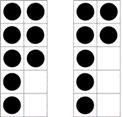Mathematics
Easy

Question

# Find 8 + 7 = ___## 1311510Hint:

## The correct answer is: 15

### Here we have to calculate the 8 + 7 = ___Firstly , we have two frame ,One have 8 dots and second have 7 dots,We have to find  +[ 7]So fill the remaining space in first frame to make it 10 , [ 8] + [2 + 5 ] ( we can write 2 + 5 = 7 )[8 + 2 ] +         ( Move 2 counters to make a ten.) + Now one frame have 10 dots other frame have 5, so add both of them to find the solutionSo 10 + 5 = 15The correct answer is 15( option (c)).

In this question ,we have to make the first number to 10 and figure is for better understanding where it has two frame and dots represent the number inside it. Make the first number to 10 and add remaining number.

### Related Questions to study#### With Turito Foundation.#### Get an Expert Advice From Turito.